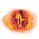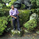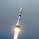TradingView5221 뷰
Hello everyone,

Quick script to check the PEG Ratio.

What is PEG Ratio?
The price/ earnings to growth ratio (PEG Ratio) is a stock's price-to-earnings (P/E) ratio divided by the growth rate of its earnings for a specified time period. The PEG ratio is used to determine a stock's value while also factoring in the company's expected earnings growth, and is thought to provide a more complete picture than the more standard P/E ratio .

A PEG Ratio greater than 1.0 means that the stock is overvalued, while below 1.0 means is is undervalued. When the PEG Ratio is exactly 1.0, then the stock is trading at fair valuation.

Formula:
PEG Ratio = (Price / EPS ) / EPS Growth

Examples:

Company A:
• Price per share = \$46
• EPS this year = \$2.09
• EPS last year = \$1.74
Company B
• Price per share = \$80
• EPS this year = \$2.67
• EPS last year = \$1.78

Company A
• P/E ratio = \$46 / \$2.09 = 22
• Earnings growth rate = (\$2.09 / \$1.74) - 1 = 20%
• PEG ratio = 22 / 20 = 1.1
Company B
• P/E ratio = \$80 / \$2.67 = 30
• Earnings growth rate = (\$2.67 / \$1.78) - 1 = 50%
• PEG ratio = 30 / 50 = 0.6

The company A is overvalued whiled the B is undervalued.

In this script an overvalued stock is considered when the PEG Ratio is above 1.1, while it is 0.9 for an undervalued stock.

Only works with Stocks.

Happy trading,
오픈 소스 스크립트

진정한 TradingView의 정신에 따라, 이 스크립트의 작성자는 스크립트를 오픈소스로 게시했기에 거래자들이 이해하고 확인할 수 있습니다. 작가님께 건배! 스크립트를 무료로 사용할 수 있지만, 게시물에서 이 코드를 재사용하는 것은 하우스룰을 따릅니다. 당신은 스크립트를 차트에 사용하기 위해 그것을 즐겨찾기 할 수 있습니다.

차트에 이 스크립트를 사용하시겠습니까?

## 코멘트Do you know of a way to calculate this "manually"? Price is a bar-by-bar change so presumably this can be calculated on a bar-by-bar basis (not just through FQ/FY financials).
응답Tomotto
@Tomotto, mine is set to 4, but I'm still getting the resolve error. Do you know how I can fix this?
응답Intermarketics
@Intermarketics, Can you tell me which stock you're trying to apply this script on?
응답Intermarketics
@Intermarketics, couldn't find anything yet. I'll continue to look
응답Intermarketics
@Intermarketics, Never mind, I solved the issue. Thanks though
응답